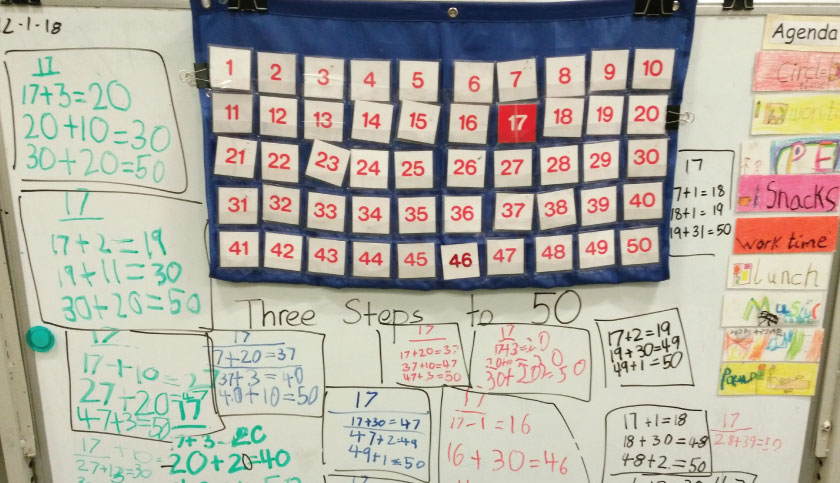# Mathematics – A Concrete and Social Learning Endeavour

Mathematics – a word that gives rise to fear in many a student’s mind the world over, but an area of study thoroughly enjoyed in the Primary School. When one reflects on various factors that have helped in creating this shift, the most obvious one it is that we make mathematical thinking real and meaningful for our young mathematicians by integrating it with other curricular areas. Engaging and meaningful experiences such as estimating and calculating the cost for going on a field trip, measuring the area of the Music Room, gathering data and then calculating the number of people present in the Assembly, constructing a compost bin for the school allows students to apply their mathematical understanding to real world, authentic situations.
Our classrooms are filled with math manipulatives, making it possible for students to visualise the abstract math concepts. Be it the understanding of numbers, place value, basic operations or concepts such as fractions (the biggest cause of math fear), manipulatives help us make math more visual and relevant. Sadly, use of such essential tools is seen as a shortcoming in students’ mathematical understanding in most schools, but not at BD Somani. Recent research done by Jo Boaler, an eminent Professor of Mathematics Education at Stanford University, points to the important role played by manipulatives (which includes our very own fingers) in enhancing and deepening mathematical understanding of young students. From a young age, students are encouraged to use objects found in their surroundings to comprehend the oneness of number one and all other numbers. In the Primary grades, students use the pattern blocks, Cuisenaire rods, base ten blocks, drawings (a very essential tool) to explore fractions, multiplication, division, percentage and various other math concepts.Guided by the work done by Sherry Parish, Kathy Richardson and Ruth Parker, Dot Talk and Number Talk have become a regular feature in our math classes. They encourage students to engage in mathematical discussions, make sense of math, model and explain their thinking, validate their responses and critique others’ responses. Talk and mathematical discourse have, thus, become a regular feature of our classrooms, giving the teacher and the students the freedom to move away from the conventional view of mathematics that laid emphasis on mastery of procedures, methods and tricks. In a conventional classroom, what makes the ‘answer’ right is the validation provided by the teacher or answers printed at the back of a textbook or shared by a ‘smart’ student. But Number Talk and Dot Talk provide an open field to all learners to make their own conjectures and justify their conjectures by providing mathematical evidence. Learning of math is not seen as an individual activity but a social activity, and the mathematical knowledge and understanding built is collectively owned both by the teachers and the students.
Underlying the mathematical discourse in the primary classrooms are the habits of the mind, disposition, thinking processes required to do the math and are similar to those used by mathematicians. At B. D. Somani, Primary School we have adopted the eight Mathematical Practices of the Common Core State Standards which promote mathematical thinking. These are not pedagogical practices but are habits of mind that mathematicians use in their work.

### The eight Mathematical Practices are:

1. Make sense of problems and persevere in solving them
2. Reason abstractly and quantitatively
3. Construct viable arguments and critique the reasoning of others
4. Model with mathematics
5. Use appropriate tools strategically
6. Attend to precision
7. Look for and make use of structure
8. Look for and express regularity in repeated reasoning
After a lot of deliberation and discussion involving mathematicians, researchers and math educators the Mathematical Practices were included in the US Common Core State Standards in Mathematics. A detailed explanation of these practices requires a whole new blog. These practices have forced us to look at math through a different lens. They’ve helped teachers to dig into their students’ understanding, the patterns they see, the connections they make and the meaning they derive while doing math. Our young learners are no longer in a race to finish the maximum number of ‘sums’ but are actively engaged, invested and completely involved in the process, enabling the teachers to help them in uncovering the curriculum. This approach decreases “math phobia” and, most importantly, enables students to be actively engaged in genuine mathematical thinking and reasoning in higher level math endeavours.

### References:

Lampert, M.; Rittenhouse, P. & Crumbaugh, C. (1996) Agreeing to disagree: Developing sociable mathematical discourse. In Olson, D. & Torrance, N. (Eds.) Handbook of Education and Human Development. Oxford, Blackwell’s Press, pp. 731-764.